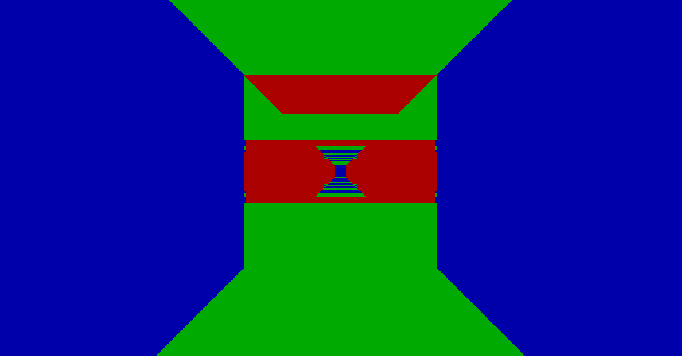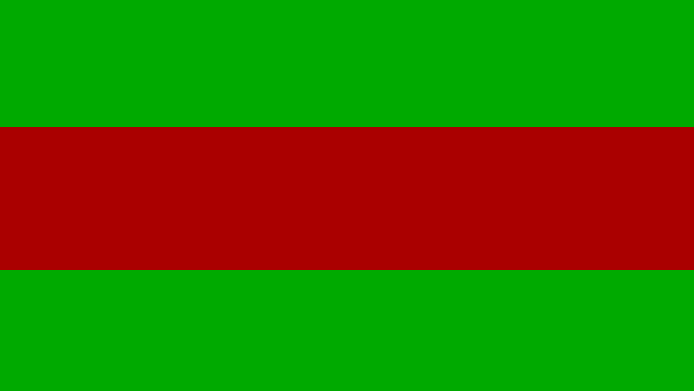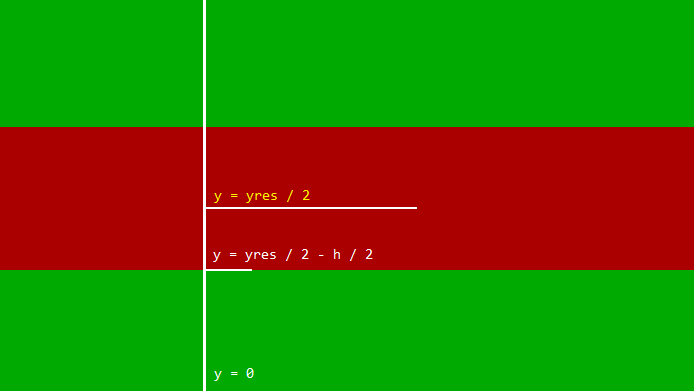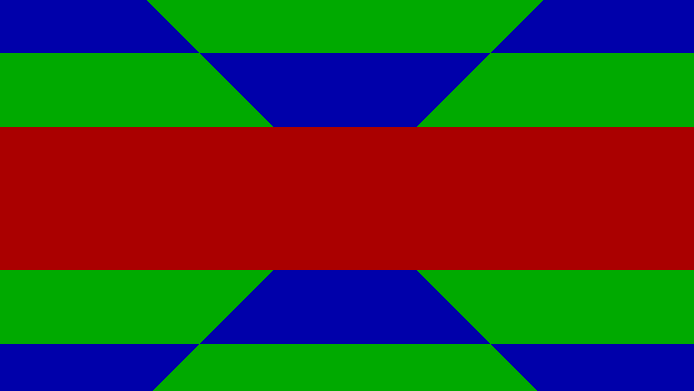GitHub Source

Raycasting howto blogs are a dime a dozen, though little about ceiling or floor casting is ever mentioned.# Wall Height

The height of the wall is calculated by taking the normal ray to the wall (in this case, the x direction), and multiplying the focal length of the field of view. The normal is clamped to a small value in case the player gets too close to the screen.

``````const float normal = ray.x < 1e-2f ? 1e-2f : ray.x;
const float size = 0.5f * focal * xres / normal;
``````

The ray is defined as the difference of the vectors of the wall hit and the player:

``````const Ray ray = sub(wall.hit.where, hero.where);
``````

The top and bottom of the wall can be found by subtracting half the size of the wall from the middle of the screen’s y-resolution.

``````const int top = (yres + size) / 2.0f;
const int bot = (yres - size) / 2.0f;
``````

When the player is parallel to a wall the following scene is rendered:# Ceiling and Floor casting

Ceiling and floor casting require a percentage of the floor in relation to the wall height:An expression for y:

``````y = yres / 2 - h / 2
``````

Where h is the size of the wall calculated earlier. With a bit of reordering a percentage (p) expression for y is simply:

``````p = -h / (2 * y - yres)
``````

As both (h) and (yres) are constant, (y) will vary and change the percentage (p). This percentage is then used to lerp the along the casted ray:The full source is here.

See pcast(). This handles the floor and ceiling casting.